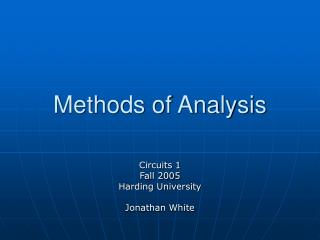DownloadDownload PresentationMethods of Analysis

# Methods of Analysis

Télécharger la présentation## Methods of Analysis

- - - - - - - - - - - - - - - - - - - - - - - - - - - E N D - - - - - - - - - - - - - - - - - - - - - - - - - - -
##### Presentation Transcript

1. Methods of Analysis Circuits 1 Fall 2005 Harding University Jonathan White

2. Outline • Nodal Analysis • Define a symbol for all unknown node voltages. • Write KCL at each node where variables occur • Using Ohm’s Law, solve resulting equations. • Mesh Currents • Set up the currents • Use KVL • Methods to solve linear equations • Substitution • Determinants • Calculator • Method from Numerical Methods

3. Nodal Analysis • Steps: • Define a voltage at every node in the circuit • Note: Some may be known, such as the source and ground • Write KCL at the nodes where the unknown voltages exist • Now, plug into these KCL equations with the unknown voltages, remembering how Ohm’s Law works. In this case, I = (VH – VL)/R, because we are writing voltages for nodes, not just resistors. Since current flows from a higher potential to a lower potential, the voltage over a resistor that is connected to 2 nodes is just VH – VL • Other current and voltage sources must be factored in to either the KCL equations or the unknown voltages. They sometimes actually make the equations easier. • Solve for the unknown voltages.

4. Nodal Analysis Example 1 Find all voltages and currents.

5. Nodal Analysis Example 2 Find Vo + Vo -

6. Mesh Currents • Steps: • Label each unknown current in each mesh, going clockwise. • A mesh is a loop which does not contain any other loops within it. • Also, write down the polarities of the currents as they go through each resistor. • Write KVL equations for each mesh. In this case, use V=I*R. When resistors are in both meshes, I=(I1-I2). • Use Ohm’s Law to express the voltages in terms of the mesh currents. • Again, you may need extra equations if there are other current/voltage sources. • Solve for the unknown currents.

7. Mesh Current Example - 1 Calculate the mesh currents.

8. Mesh Current Example - 2 Find the current through the 1 ohm R

9. Methods of Solving Sets of Equations • Calculator • rref function • solve function • Linear Algebra • Substitution • Graphing • Euclid’s Method